up

# Quantum Computing. Less Formulas — More Understanding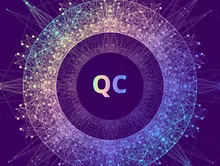Start date will be announced later
• English

course language

• 6 weeks

course duration

• от 4 до 6 часов в неделю

needed to educate

• 2 credit points

This is yet one more introductory course on quantum computing. Here I concentrate more on how the mathematical model of quantum computing grows out from physics and experiment, while omitting most of the formulas (when possible) and rigorous proofs.

On the first week I try to explain in simple language (I hope) where the computational power of a quantum computer comes from, and why it is so hard to implement it. To understand the materials of this week you don't need math above the school level.

Second and third weeks are about the mathematical model of quantum computing, and how it is justified experimentally. Some more math is required here. I introduce the notion of a linear vector space, discuss some simple differential equations and use complex numbers.

The forth week is dedicated to the mathematical language of quantum mechanics. You might need this if you want to dig deeper into subject, however I touch only the tip of the iceberg here.

On the week 5 I finally introduce some simple quantum algorithms for cryptography and teleportation.

## Format

The form of education is online. Weekly classes will include watching thematic video lectures, studying additional materials and completing tests with automated verification of results. All assignments must be completed to obtain a certificate.

## Course program

Two Basic Questions

The Origins of the Mathematical Model. Part 1

The Origins of the Mathematical Model. Part 2

The Language of Quantum Mechanics

Quantum Cryptography and Teleportation

## Education results

• Comparing classical and quantum computations
• Understanding of quantum parallelism
• Construction of quantum logic algorithms
• Designing of quantum computational models
• Computation with qubits
• Computation with continuous variables
• Quantum error correction

## Education directions

The course is aimed at the formation of general learning competencies for Bachelor’s and Specialists’ programs, as well as other competencies included in the education program.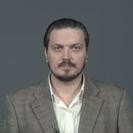### Сысоев Сергей Сергеевич

Кандидат физико-математических наук
Position: доцент

## Similar courses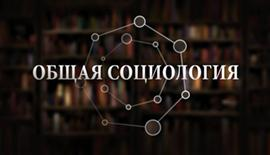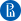НИУ ВШЭ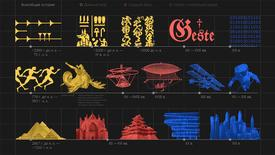СПбГУ